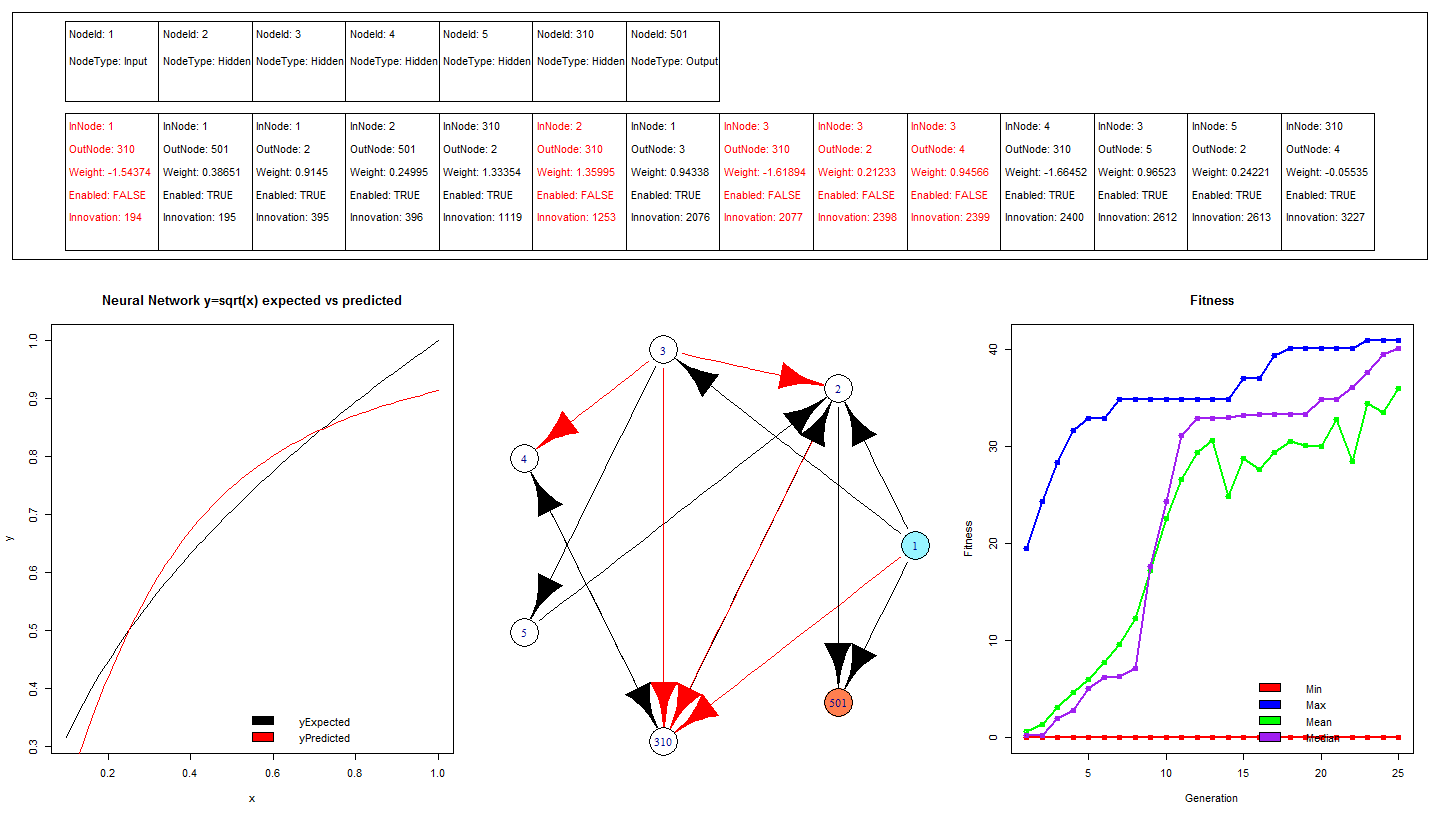# RNeat – Square Root Neural Net trained using Augmenting Topologies – Simple Example

A simple tutorial demonstrating how to train a neural network to square root numbers using a genetic algorithm that searches through the topological structure space. The algorithm is called NEAT (Neuro Evolution of Augmenting Topologies) available in the RNeat package (not yet on CRAN).

The training is very similar to other machine learning / regression packages in R. The training function takes a data frame and a formula. The formula is used to specify what columns in the data frame are the dependent variables and which are the explanatory variable. The code is commented and should be simple enough for new R users.The performance of the network can be seen in the bottom left chart of the image above, there is considerable differences between the expected output and the actual output. It is likely that with more training the magnitude of these errors will reduce, it can be seen in the bottom right chart that the maximum, mean and median fitness are generally increasing with each generation.

?View Code RSPLUS
 ```install.packages("devtools") library("devtools") install_github("RNeat","ahunteruk") #Install from github as not yet on CRAN library("RNeat")   #Generate traing data y = sqrt(x) trainingData <- as.data.frame(cbind(sqrt(seq(0.1,1,0.1)),seq(0.1,1,0.1))) colnames(trainingData) <- c("y","x")   #Train the neural network for 5 generations, and plot the fitness rneatsim <- rneatneuralnet(y~x,trainingData,5) plot(rneatsim)   #Continue training the network for another 5 generations rneatsim <- rneatneuralnetcontinuetraining(rneatsim,20) plot(rneatsim)   #Construct some fresh data to stick through the neural network and hopefully get square rooted liveData <- as.data.frame(seq(0.1,1,0.01)) colnames(liveData) <- c("x")   liveDataExpectedOutput <- sqrt(liveData) colnames(liveDataExpectedOutput) <- "yExpected"   #Pass the data through the network results <- compute(rneatsim,liveData)   #Calculate the difference between yPred the neural network output, and yExpected the actual square root of the input error <- liveDataExpectedOutput[,"yExpected"] - results[,"yPred"] results <- cbind(results,liveDataExpectedOutput,error)   dev.new() layout(matrix(c(3,3,3,1,4,2), 2, 3, byrow = TRUE),heights=c(1,2)) plot(x=results[,"x"],y=results[,"yExpected"],type="l", main="Neural Network y=sqrt(x) expected vs predicted",xlab="x",ylab="y") lines(x=results[,"x"],y=results[,"yPred"],col="red",type="l") legend(x='bottomright', c('yExpected','yPredicted'), col=c("black","red"), fill=1:2, bty='n') plot(rneatsim) plot(rneatsim\$simulation)```

## 6 thoughts on “RNeat – Square Root Neural Net trained using Augmenting Topologies – Simple Example”

1.spring roll on said:

Hey gekkoquant, Thank you for considering my suggestion and its real awesome.

2.spring roll on said:

Hello gekkoquant, I am trying out your package in my system. I am using some more data like this
rneatsim <- rneatneuralnet(y~x1+x2+x3…Xn,trainingData,5)

its working fine but the process is overheating my system. I know its not the fault of your package but of my system.

For my custom functions I usually add a system sleep for 3 to 5 seconds[ Sys.sleep(3)] at the end of a loop. The looping takes more time to complete but my system remains in ideal temperature thus preventing system overheating.

Can you add a similar custom sleep as a parameter to your rneatneuralnet() function. so that users can add sleep time manually?

Thank you

3.spring roll on said:

Once more hello gekko quant,
Sorry if I am causing any inconvenience but I have to say this that I tried my own method using trace function trace(“rneatneuralnetcontinuetraining”,edit=TRUE)
Sys.sleep(6)
at the end of the for loop but my solution is not working. I have see this link mathworks.com/help/nnet/ug/checkpoint-saves-during-neural-network-training.html about auto saving NN; this is just a suggestion but is it possible to add an auto save option let say every 100 iterations or every one generation to RNeat. This way we can train and save(rda/Rdata) a neural network at any interval of time
say train for 5 minutes save the NN and come back tomorrow load the NN from file start from the last position?

Any way keep up the good work

4.spring roll on said:

This is my last comment for this post so please bare with me :). I have solved the problem you already gave a solution for my problem and my dumb mind didnt saw it first. instead of training in a single run now I am training NEAT model in multiple runs like this

save(rneatsim,file=”model.rda”)
rneatsim <- rneatneuralnetcontinuetraining(rneatsim,5)

this way only once in a day I have to run it and improve the model further.

Once more thank you very much. Now I will not bother you(most probably)

5.Senda on said:

Hi
Thank you for your great work with R!
Please I have a question, I tried your program on data about firms, two tables with 52 observations for 6 variables.
Everything works fine, except when testing the neural network I always have the same error message
“Error in `[.data.frame`(result\$data, , result\$rneatsim\$inputs\$model.list\$variables) :
undefined columns selected”
This is what I did:

chemin <-choose.files()
chemin <-choose.files()
edit(testdata)
install.packages("devtools")
library("devtools")
install_github("RNeat","ahunteruk") #Install from github as not yet on CRAN
library("RNeat")
rneatsim <- rneatneuralnet(y~a+b+c+d+e,trainingdata,5)
plot(rneatsim)
rneatsim <- rneatneuralnetcontinuetraining(rneatsim,20)
plot(rneatsim)
inputs <- as.data.frame(testdata[,2:6])
edit(inputs)
results <- compute(rneatsim, inputs)

I always tried this:
results <- compute(rneatsim, f+g+h+i+j)

results <- compute(rneatsim,testdata[,2:6])

results <- compute(rneatsim, testdata\$f+testdata\$g+testdata\$h+testdata\$i+testdata\$j)

inputs <- testdata\$f+testdata\$g+testdata\$h+testdata\$i+testdata\$j
results <- compute(rneatsim,inputs)

inputstest <- testdata[,2:6]
results <- compute(rneatsim, inputstest)

But I'm always getting the same error message!